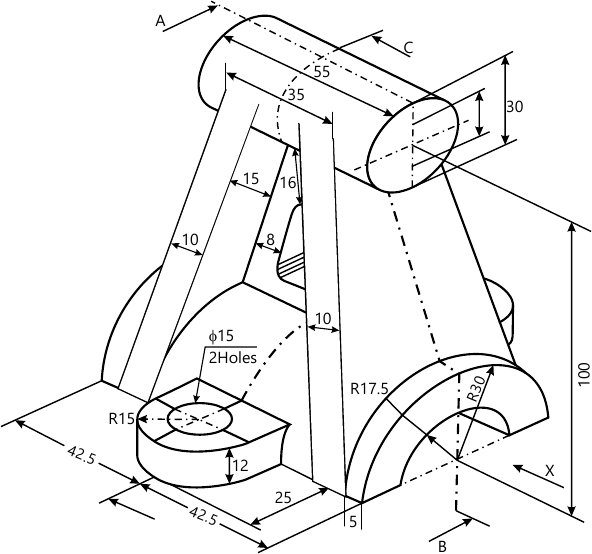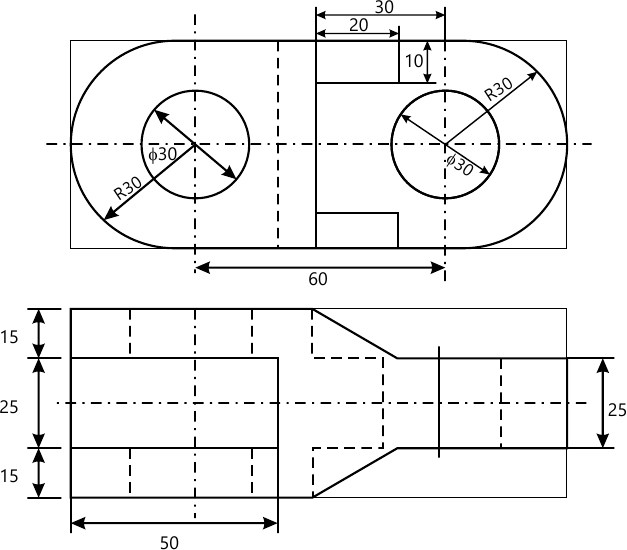MORE IN Engineering Graphics
GTU First Year Engineering (Semester 1)
Engineering Graphics
December 2014
Total marks: --
Total time: --
INSTRUCTIONS
(1) Assume appropriate data and state your reasons
(2) Marks are given to the right of every question
(3) Draw neat diagrams wherever necessary

1 (a) (i) Name the solid formed by revolving right angle triangle with one of its perpendicular side fixed.
(a) Cone
(b) Tetrahedron
(c) Cylinder
(d) Octahedron
1 M
1 (a) (ii) To draw the leader line, which type of the following line is used?
(a) Continuous thick line
(b) Continuous thin wavy line
(c) Long chain thin line
(d) Continuous thin line
1 M
1 (a) (iii) If the object lies in the second quadrant, its position with respect to reference plane will be
(a) In front of V.P. and above H.P.
(b) Behind V.P. and below H.P.
(c) In front of V.P. and below H.P.
(d) Behind V.P. and above H.P.
1 M
1 (a) (iv) When the drawing are drawn smaller than the actual size of object then scale is known as
(a) Reduced Scale
(b) Full Scale
(c) Enlarged Scale
(d) None of Above
1 M
1 (a) (v) A curved traced out by a point which moves uniformly both about the centre and at the same time away or towards the centre is known as
(a) Involute
(b) Cycloid
(c) Archemedian spiral
(d) None of above
1 M
1 (a) (vi) The isometric view of a vertical line is represented at an angle of ____ in front view and having a length _________ the original length of line.
(a) 30°, Same as
(b) 30°, Less that
(c) 90°, Same as
(d) 90°, Less than
1 M
1 (a) (vii) In a third angle projection method, right hand side view of an object is drawn ___________ front view.
(a) Left side of
(b) Rear side of
(c) Right side of
(d) None of above
1 M
1 (b) (i) If the 10 m length is represented as 1 mm on the map then representative fraction is
(a) 1/100
(b) 1/10
(c) 1/1000
(d) None of above
1 M
1 (b) (ii) Fourth angle projection is not used because
(a) Front view is above reference line and top view is below reference line
(b) Top view is above reference line and front view is below reference line
(c) Front view and top view both overlap on each other and below reference line
(d) Front view and top view both overlap on each other and above the reference line
1 M
1 (b) (iii) When the cone, resting on base on V.P., is cut by section plane parallel to V.P. then the true shape is __________ and can be seen in __________ view.
(a) Circle, Front
(b) Ellipse, To (c) Ellipse, Front
p
(d) Circle, Top
1 M
1 (b) (iv) The eccentricity of which of the following curve is greater than one?
(a) Ellipse
(b) Hyperbola
(c) Parabola
(d) None of above
1 M
1 (b) (v) The isometric projection of 90 mm line is ___________ mm.
(a) 30×(6)(1/2)
(b) 30×(2)(1/2)
(c) 30×(3)(1/2)
(d) None of above.
1 M
1 (b) (vi) For the third angle projection method, Which of the following is correct?
(a) Observer-Object-Plane
(b) Observer-Plane-Object
(c) (a) and (b) both
(d) None of above
1 M
1 (b) (vii) When the front view of line having a length less than the original length then which of the following is correct?
(a) Line is inclined to H.P.
(b) Line is inclined to V.P.
(c) Line is inclined to both H.P. and V.P.
(d) (b) and (c) both
1 M

2 (a) The foci of an ellipse are 110 mm apart. The minor axis is 70 mm long. Determine the length of major axis and draw half ellipse by rectangle method and other half by concentric circle method.
7 M
2 (b) A line PQ, 65 mm long, is inclined to H.P. by 30° and inclined to V.P. by 45°. The end P is 20 mm below H.P. and 25 mm behind V.P. Point Q is in fourth quadrant. Draw its projections and find the position of the point Q.
7 M

3 (a) The front view and top view of a line MN is inclined at an angle of 30° and 40° respectively. The front view of line MN measures 50 mm. Point M is 15 mm above H.P. and 10 mm in front of V.P. Draw the projections of line MN and find the true length of line MN.
7 M
3 (b) A square plate of side 40 mm is rest on one of its corner on H.P. with diagonal horizontal and inclined at 50° to V.P. The Plate is seen as a rhombus in plan with one of its diagonals measuring 30 mm. Draw the projections
7 M

4 (a) A regular pentagonal plate is resting in V.P. on one of its sides with surface making an angle 45° with V.P. The side on which it rests on V.P. makes 60° with H.P. Draw the projections
7 M
4 (b) A frustum of hexagonal pyramid, side of base 30 mm and height 60 mm, is cutting from a pyramid of height 80 mm, is standing upright with base on H.P. and axis parallel to V.P. It is cut by section plane making an angle of 50° to V.P. and remaining 20 mm away from the axis. Draw top view, sectional front view, sectional left hand side view and true shape of section.
7 M

5 (a) Draw the projection of a cone, base 44 mm diameter and axis 50 mm long, when it is resting on the H.P. on a point of its base circle with the axis making an angle of 45° with H.P. and 30° with V.P.
7 M
5 (b) A circle of 50 mm diameter rolls along a straight line without slipping. Trace the path of a point on the circumference of the rolling circle for one complete revolution. Name the curve.
7 M

6 (a) On a map of a state, 1 cm represents 5 kms. Construct a plain scale long enough to measure a distance between two city 100 kms far from each other.
3 M
6 (b) Using the first angle projection method, draw the following view for the figure: - 1. Give the dimensions using the Aligned dimensioning method.
(i) Sectional front view by taking section along C -D
(ii) Sectional left hand side view by taking section along A - B
(iii) Top view7 M

7 (a) Construct a diagonal scale of representative fraction=(1/36) showing yard, foot and inch. Scale should be long enough to measure 5 yard.
4 M
7 (b) Draw the isometric view for the figure:-2.10 M

More question papers from Engineering Graphics